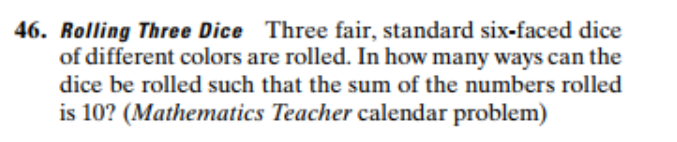# 46. Rolling Three Dice Three fair, standard six-faced diceof different colors are rolled. Inhow many ways can thedice be rolled such that the sum of the numbers rolledis 10? (Mathematics Teacher calendar problem)

Question
3 viewshelp_outlineImage Transcriptionclose46. Rolling Three Dice Three fair, standard six-faced dice of different colors are rolled. Inhow many ways can the dice be rolled such that the sum of the numbers rolled is 10? (Mathematics Teacher calendar problem) fullscreen
check_circle

Step 1

Given data

3 fair, six-faced dice are rolled

18 possible ways to get a sum of 10

(1,5,4), (4,5,1), (5,4,1) = 1+5+4 =10

(1,6,3), (3,6,1), (6,3,1) = 1+6+3 =10

(2,2,6), (2,6,2), (6,2,2) ...

### Want to see the full answer?

See Solution

#### Want to see this answer and more?

Solutions are written by subject experts who are available 24/7. Questions are typically answered within 1 hour.*

See Solution
*Response times may vary by subject and question.
Tagged in

### Basic Probability